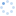# 并联电阻的等效计算公式

0
[导读] 　　并联电阻的等效计算公式为： 　　1R =1R1 +1R2 +…+1Rn (1) 　　使用该公式时，有两种情况计算比较方便： 　　① 并联的电阻比较少时，如两个电阻并联时，一

并联电阻的等效计算公式为：

1R =1R1 +1R2 +…+1Rn (1)

使用该公式时，有两种情况计算比较方便：

① 并联的电阻比较少时，如两个电阻并联时，一般都是直接由公式R=R1×R2R1+R2 求得等效电阻 ;

② 当并联的n个电阻阻值相等时，等效电阻为 R=R1n 。

但当多个电阻并联且电阻值又都不相等时，计算就比较烦琐，为此，本文对公式(1)进行了变形，使多个电阻的并联计算变得简化。

将公式(1)变形可得：

R= 1 1R1 +1R2 +…+1Rn = Ri RiR1 +RiR2 +…+RiRn = Ri K1+K2+…+Kn (2)

其中K1=RiR1 ，K2=RiR2 ，… Kn=RiRn ，Ri为n个并联电阻中的一个，Ri的选择可遵循如下的规则：

① 选能被其它电阻整除的一个电阻作Ri

例1 有三个电阻并联，R1=3Ω，R2=6Ω，R3=18Ω，则选电阻R3作为被除电阻Ri，即： K1=183 =6，K2=186 =3，K3=1818 =1

等效电阻 R=Ri K1+K2+K3 = 18 6+3+1 =2Ω

②当找不到一个电阻能被其它电阻整除时，选阻值最大的电阻作为被除电阻Ri 。

例2 三个电阻R1=8Ω，R2=10Ω，R3=12Ω并联，则选阻值最大的电阻R3=12Ω作为被除电阻Ri，计算就比较方便，此时有:

K1=128 =1.5，K2=1210 =1.2，K3=1212 =1

等效电阻 R=Ri K1+K2+K3 = 12 1.5+1.2+1 =12 3.7 =3.24Ω

当然,也可以任选一个电阻作为被除电阻Ri，但与选择阻值最大的电阻作为被除电阻时相比，计算时小数增多,增加了烦琐程度，甚至影响计算精度.

例如,例2中，选8Ω的电阻作为被除电阻Ri，则有：

K1=88 =1，K2=810 =0.8，K3=812 =0.67

得等效电阻 R=Ri K1+K2+K3 = 8 1+0.8+0.67 =8 2.47 =3.23Ω

可见,计算比上例烦琐,精度也有所降低.

③也可以选择n个电阻之外的任意一个阻值作被除电阻，这个电阻可以选成能被所有的n个电阻整除，这样计算更方便。

例如，例2中的三个电阻R1=8Ω，R2=10Ω，R3=12Ω并联时，可选一个能被三个电阻都整除的数值作被除电阻值，如选120Ω，则有：

K1=1208 =15，K2=12010 =12，K3=12012 =10

等效电阻

R= Ri K1+K2+K3 = 120 15+12+10 = 120 37 =3.24Ω

结果与例2一致，但计算中少了小数，更容易被接受。

公式(2)的物理意义，就是把所有的电阻都折算成电阻Ri的并联，共折算成K1+K2+…+Kn 个Ri的并联，如上述例1中把所有的电阻都折算成18Ω电阻的并联,将3Ω看作是6个18Ω的电阻并联，6Ω的电阻可看作3个18Ω的电阻并联。上述例2中把所有的电阻都折算成8Ω电阻的并联,10Ω电阻可看作0.8个8Ω的电阻并联,12Ω可看作0.67个8Ω的电阻并联.其中0.8个8Ω的电阻可以这样理解,将8Ω的电阻纵向剖成10份,每份的截面积是原来的十分之一,电阻是原来的十倍(80Ω),取其中的8份并联,即为0.8个8Ω的电阻并联.

综上所述，运用公式(2)计算等效电阻，比公式(1)简单，尤其是当并联的电阻较多时，分解了难点，计算显得更方便了。

## 用户评论0 条评论

### 精品文章• 型 号
• 产品描述